# Third member

Determine the third member of the AP if a4=93, d=7.5.

Correct result:

a3 =  85.5

#### Solution:

$a_{4}=93 \ \\ d=7.5 \ \\ a_{3}=a_{4}-d=93-7.5=\dfrac{ 171 }{ 2 }=85.5$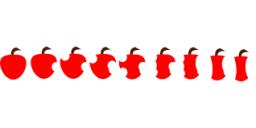We would be pleased if you find an error in the word problem, spelling mistakes, or inaccuracies and send it to us. Thank you!## Next similar math problems:

• Fifth memberDetermine the fifth member of the arithmetic progression, if the sum of the second and fifth members equal to 73, and difference d = 7.
• Quotient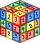Determine the quotient and the second member of the geometric progression where a3=10, a1+a2=-1,6 a1-a2=2,4.
• Arithmetic progression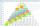In some AP applies: 5a2 + 7a5 = 90 s3 = 12 Find the first member a =? and difference d = ?
• Difference AP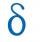Calculate the difference of arithmetic progression if the sum of its first 19 members Sn = 8075 and the first member is a1 = 20
• Determine AP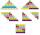Determine the difference of the arithmetic progression if a3 = 7, and a4 + a5 = 71
• HP - harmonic progressionDetermine the 8th term of the harmonic progression 2, 4/3, 1,…
• Geometric seqFind the third member of geometric progression if a1 + a2 = 36 and a1 + a3 = 90. Calculate its quotient.
• GP - three membersThe second and third of a geometric progression are 24 and 12(c+1) respectively, given that the sum of the first three terms of progression is 76 determine value of c
• GP membersThe geometric sequence has 10 members. The last two members are 2 and -1. Which member is -1/16?
• Gp - 80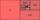Sum of the first four members of a geometric progression is 80. Determine they if we know that the fourth member is nine times greater than the second.
• AP members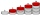What is the value of x2, x3, x4, x5…of the terms (of arithmetic progression) when x1 = 8 and x6 = 20?
• Negative differenceFind four arithmetic progression members between 7 and -6.
• Q of GP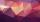Calculate quotient of geometric progression if a1=5 a a1+a2=12.
• AP - basicsDetermine first member and differentiate of the the following sequence: a3-a5=24 a4-2a5=61
• HP - harmonic progression 2Compute the 16th term of the HP if the 6th and 11th term of the harmonic progression are 10 and 18 respectively.
• Right-angled triangle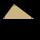Determine the content of a right triangle whose side lengths form successive members of an arithmetic progression and the radius of the circle described by the triangle is 5 cm.
• Insert 3Insert five arithmetic progression members between -7 and 3/2.